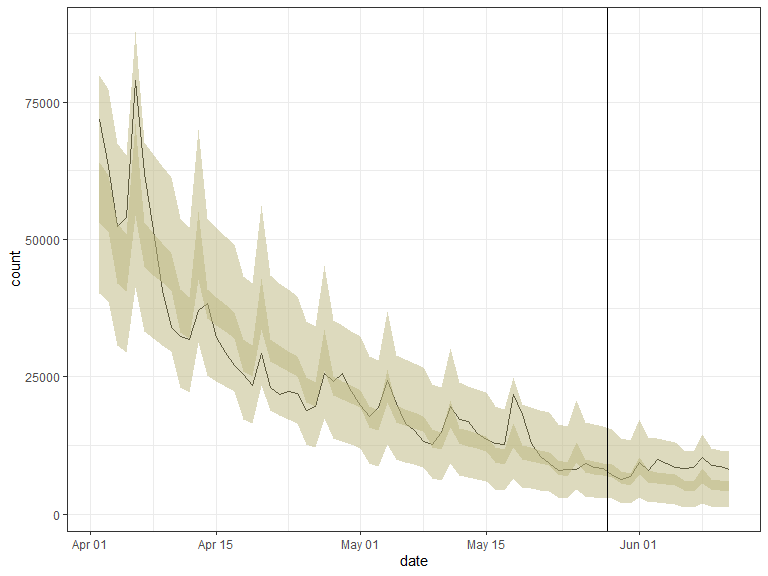trendeval aims to provide a coherent interface for evaluating models fit with the trending package. Whilst it is useful in an interactive context, it’s main focus is to provide an intuitive interface on which other packages can be developed (e.g. trendbreaker).

## Installing the package

You can install the stable version of this package from CRAN with:

install.packages("trendeval")

The development version can be installed from GitHub with:

if (!require(remotes)) {
install.packages("remotes")
}
remotes::install_github("reconverse/trendeval", build_vignettes = TRUE)

## Model selection example

library(dplyr)      # for data manipulation
library(outbreaks)  # for data
library(trending)   # for trend fitting
library(trendeval)  # for model selection

data(covid19_england_nhscalls_2020)

# define a selection of model in a named list
models  <- list(
simple = lm_model(count ~ day),
glm_poisson = glm_model(count ~ day, family = "poisson"),
glm_poisson_weekday = glm_model(count ~ day + weekday, family = "quasipoisson"),
glm_quasipoisson = glm_model(count ~ day, family = "poisson"),
glm_quasipoisson_weekday = glm_model(count ~ day + weekday, family = "quasipoisson"),
glm_negbin = glm_nb_model(count ~ day),
glm_negbin_weekday = glm_nb_model(count ~ day + weekday),
will_error = glm_nb_model(count ~ day + nonexistant)
)

# select 8 weeks of data (from a period when the prevalence was decreasing)
last_date <- as.Date("2020-05-28")
first_date <- last_date - 8*7
pathways_recent <-
covid19_england_nhscalls_2020 %>%
filter(date >= first_date, date <= last_date) %>%
group_by(date, day, weekday) %>%
summarise(count = sum(count), .groups = "drop")

# split data for fitting and prediction
dat <-
pathways_recent %>%
group_by(date <= first_date + 6*7) %>%
group_split()
fitting_data <- dat[]
pred_data <- select(dat[], date, day, weekday)

# assess the models using the evaluate_resampling
results <-
models %>%
evaluate_resampling(fitting_data, metric = "rmse") %>%
summary
results
#> # A tibble: 8 × 5
#>   model_name               metric value splits_averaged nas_removed
#>   <chr>                    <chr>  <dbl>           <dbl>       <dbl>
#> 1 glm_negbin               rmse   5238.              43           0
#> 2 glm_negbin_weekday       rmse   5224.              43           0
#> 3 glm_poisson              rmse   5193.              43           0
#> 4 glm_poisson_weekday      rmse   5166.              43           0
#> 5 glm_quasipoisson         rmse   5193.              43           0
#> 6 glm_quasipoisson_weekday rmse   5166.              43           0
#> 7 simple                   rmse   6903.              43           0
#> 8 will_error               rmse    NaN               43          43

### Example of how this output could then be used

library(tidyr)      # for data manipulation
library(purrr)      # for data manipulation
library(ggplot2)    # for plotting

# Pull out the model with the lowest RMSE
best_by_rmse <-
results %>%
slice_min(value) %>%
select(model_name) %>%
pluck(1,1) %>%
pluck(models, .)

# Now let's look at the following 14 days as well
new_dat <-
covid19_england_nhscalls_2020 %>%
filter(date > "2020-05-28", date <= "2020-06-11") %>%
group_by(date, day, weekday) %>%
summarise(count = sum(count), .groups = "drop")

all_dat <- bind_rows(pathways_recent, new_dat)

out <-
best_by_rmse %>%
fit(pathways_recent) %>%
predict(all_dat) %>%
pluck(1)
out
#> <trending_prediction> 71 x 9
#>    date         day weekday      count estimate lower_ci upper_ci lower_pi upper_pi
#>    <date>     <int> <fct>        <int>    <dbl>    <dbl>    <dbl>    <dbl>    <dbl>
#>  1 2020-04-02    15 rest_of_week 71917   58301.   53120.   63988.    40268    79935
#>  2 2020-04-03    16 rest_of_week 63365   56280.   51398.   61625.    38771    77291
#>  3 2020-04-04    17 weekend      52412   47107.   41966.   52878.    30644    67450
#>  4 2020-04-05    18 weekend      54014   45474.   40576.   50962.    29459    65284
#>  5 2020-04-06    19 monday       78996   62206.   54362.   71181.    41350    87955
#>  6 2020-04-07    20 rest_of_week 62026   48871.   45017.   53054.    33258    67650
#>  7 2020-04-08    21 rest_of_week 51692   47176.   43540.   51116.    31991    65458
#>  8 2020-04-09    22 rest_of_week 40797   45540.   42108.   49253.    30765    63346
#>  9 2020-04-10    23 rest_of_week 33946   43961.   40718.   47463.    29580    61313
#> 10 2020-04-11    24 weekend      32269   36796.   33092.   40916.    23141    53834
#> # … with 61 more rows

# plot output
ggplot(out, aes(x = date, y = count)) +
geom_line() +
geom_ribbon(mapping = aes(x = date, ymin = lower_ci, ymax = upper_ci),
data = out, alpha = 0.5, fill = "#BBB67E") +
geom_ribbon(mapping = aes(x = date, ymin = lower_pi, ymax = upper_pi),
data = out, alpha = 0.5, fill = "#BBB67E") +
geom_vline(xintercept = as.Date("2020-05-28") + 0.5) +
theme_bw()# Resources

## Getting help online

Bug reports and feature requests should be posted on github using the issue system. All other questions should be posted on the RECON slack channel see https://www.repidemicsconsortium.org/forum/ for details on how to join.